GATE  >  Civil Engineering - (CE) 2018 GATE Paper (Practice Test - 2)

# Civil Engineering - (CE) 2018 GATE Paper (Practice Test - 2)

Test Description

## 65 Questions MCQ Test GATE Past Year Papers for Practice (All Branches) | Civil Engineering - (CE) 2018 GATE Paper (Practice Test - 2)

Civil Engineering - (CE) 2018 GATE Paper (Practice Test - 2) for GATE 2023 is part of GATE Past Year Papers for Practice (All Branches) preparation. The Civil Engineering - (CE) 2018 GATE Paper (Practice Test - 2) questions and answers have been prepared according to the GATE exam syllabus.The Civil Engineering - (CE) 2018 GATE Paper (Practice Test - 2) MCQs are made for GATE 2023 Exam. Find important definitions, questions, notes, meanings, examples, exercises, MCQs and online tests for Civil Engineering - (CE) 2018 GATE Paper (Practice Test - 2) below.
Solutions of Civil Engineering - (CE) 2018 GATE Paper (Practice Test - 2) questions in English are available as part of our GATE Past Year Papers for Practice (All Branches) for GATE & Civil Engineering - (CE) 2018 GATE Paper (Practice Test - 2) solutions in Hindi for GATE Past Year Papers for Practice (All Branches) course. Download more important topics, notes, lectures and mock test series for GATE Exam by signing up for free. Attempt Civil Engineering - (CE) 2018 GATE Paper (Practice Test - 2) | 65 questions in 180 minutes | Mock test for GATE preparation | Free important questions MCQ to study GATE Past Year Papers for Practice (All Branches) for GATE Exam | Download free PDF with solutions
 1 Crore+ students have signed up on EduRev. Have you?
Civil Engineering - (CE) 2018 GATE Paper (Practice Test - 2) - Question 1

### “His face ___________ with joy when the solution of the puzzle was _______ to him.” The words that best fill the blanks in the above sentence are

Civil Engineering - (CE) 2018 GATE Paper (Practice Test - 2) - Question 2

### “Although it does contain some pioneering ideas, one would hardly characterize the work as _________.” The word that best fills the blank in the above sentence is

Civil Engineering - (CE) 2018 GATE Paper (Practice Test - 2) - Question 3

###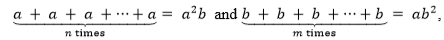where a, b, n and m are natural numbers. What is the value of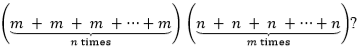Civil Engineering - (CE) 2018 GATE Paper (Practice Test - 2) - Question 4

A three-member committee has to be formed from a group of 9 people. How many such distinct committees can be formed?

Civil Engineering - (CE) 2018 GATE Paper (Practice Test - 2) - Question 5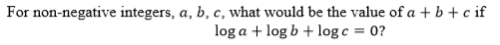Civil Engineering - (CE) 2018 GATE Paper (Practice Test - 2) - Question 6

In manufacturing industries, loss is usually taken to be proportional to the square of the deviation from a target. If the loss is Rs. 4900 for a deviation of 7 units, what would be the loss in Rupees for a deviation of 4 units from the target?

Civil Engineering - (CE) 2018 GATE Paper (Practice Test - 2) - Question 7

A faulty wall clock is known to gain 15 minutes every 24 hours. It is synchronized to the correct time at 9 AM on 11th July. What will be the correct time to the nearest minute when the clock shows 2 PM on 15th July of the same year?

Civil Engineering - (CE) 2018 GATE Paper (Practice Test - 2) - Question 8

The annual average rainfall in a tropical city is 1000 mm. On a particular rainy day (24-hour period), the cumulative rainfall experienced by the city is shown in the graph. Over the 24-hour period, 50% of the rainfall falling on a rooftop, which had an obstructionfree area of 50 m2, was harvested into a tank. What is the total volume of water collected in the tank in liters?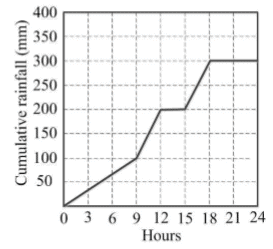Civil Engineering - (CE) 2018 GATE Paper (Practice Test - 2) - Question 9

Given that log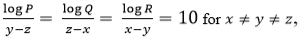what is the value of the product PQR?

Civil Engineering - (CE) 2018 GATE Paper (Practice Test - 2) - Question 10

Each of the letters in the figure below represents a unique integer from 1 to 9. The letters are positioned in the figure such that each of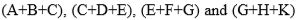is equal to 13. Which integer does E represent?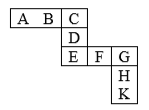Civil Engineering - (CE) 2018 GATE Paper (Practice Test - 2) - Question 11

The solution of the equation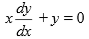passing through the point (1,1) is

Civil Engineering - (CE) 2018 GATE Paper (Practice Test - 2) - Question 12

The graph of a function f(x) is shown in the figure.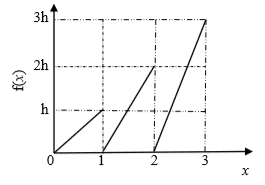For f(x) to be a valid probability density function, the value of h is

Civil Engineering - (CE) 2018 GATE Paper (Practice Test - 2) - Question 13

A probability distribution with right skew is shown in the figure.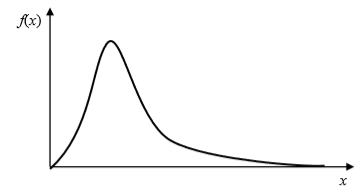The correct statement for the probability distribution is

Civil Engineering - (CE) 2018 GATE Paper (Practice Test - 2) - Question 14

All the members of the planar truss (see figure), have the same properties in terms of area of cross-section (A) and modulus of elasticity (E).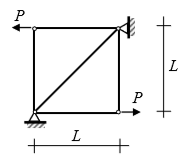For the loads shown on the truss, the statement that correctly represents the nature of forces in the members of the truss is:

Civil Engineering - (CE) 2018 GATE Paper (Practice Test - 2) - Question 15

The setting time of cement is determined using

Civil Engineering - (CE) 2018 GATE Paper (Practice Test - 2) - Question 16

A structural member subjected to compression, has both translation and rotation restrained at one end, while only translation is restrained at the other end. As per IS 456 : 2000, the effective length factor recommended for design is

Civil Engineering - (CE) 2018 GATE Paper (Practice Test - 2) - Question 17

A vertical load of 10 kN acts on a hinge located at a distance of L/4 from the roller support Q of a beam of length L (see figure).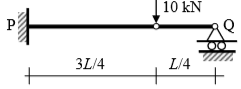The vertical reaction at support Q is

Civil Engineering - (CE) 2018 GATE Paper (Practice Test - 2) - Question 18

A flownet below a dam consists of 24 equipotential drops and 7 flow channels. The difference between the upstream and downstream water levels is 6 m. The length of the flow line adjacent to the toe of the dam at exit is 1 m. The specific gravity and void ratio of the soil below the dam are 2.70 and 0.70, respectively. The factor of safety against piping is

Civil Engineering - (CE) 2018 GATE Paper (Practice Test - 2) - Question 19

The contact pressure and settlement distribution for a footing are shown in the figure.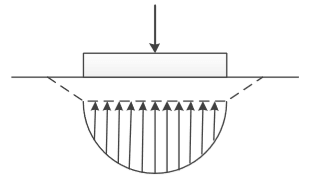The figure corresponds to a

Civil Engineering - (CE) 2018 GATE Paper (Practice Test - 2) - Question 20

Which one of the following statements is NOT correct?

Civil Engineering - (CE) 2018 GATE Paper (Practice Test - 2) - Question 21

The clay mineral, whose structural units are held together by potassium bond is

Civil Engineering - (CE) 2018 GATE Paper (Practice Test - 2) - Question 22

Dupuit’s assumptions are valid for

Civil Engineering - (CE) 2018 GATE Paper (Practice Test - 2) - Question 23

For a given discharge in an open channel, there are two depths which have the same specific energy. These two depths are known as

Civil Engineering - (CE) 2018 GATE Paper (Practice Test - 2) - Question 24

As per IS 10500:2012, for drinking water in the absence of alternate source of water, the permissible limits for chloride and sulphate, in mg/L, respectively are

Civil Engineering - (CE) 2018 GATE Paper (Practice Test - 2) - Question 25

In the figures, Group I represents the atmospheric temperature profiles (P, Q, R and S) and Group II represents dispersion of pollutants from a smoke stack (1, 2, 3 and 4). In the figures of Group I, the dashed line represents the dry adiabatic lapse rate, whereas the horizontal axis represents temperature and the vertical axis represents the altitude.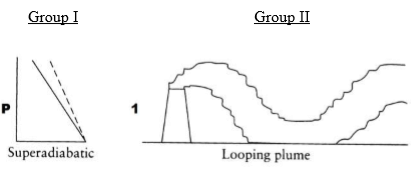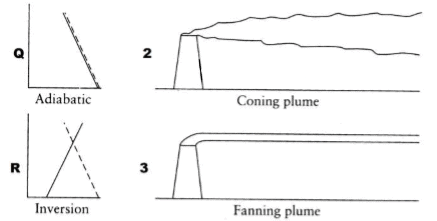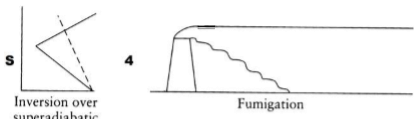The correct match is

Civil Engineering - (CE) 2018 GATE Paper (Practice Test - 2) - Question 26

Peak Hour Factor (PHF) is used to represent the proportion of peak sub-hourly traffic flow within the peak hour. If 15-minute sub-hours are considered, the theoretically possible range of PHF will be

Civil Engineering - (CE) 2018 GATE Paper (Practice Test - 2) - Question 27

As per IRC:37-2012, in order to control subgrade rutting in flexible pavements, the parameter to be considered is

Civil Engineering - (CE) 2018 GATE Paper (Practice Test - 2) - Question 28

The initial concavity in the load-penetration curve of a CBR test is NOT due to

Civil Engineering - (CE) 2018 GATE Paper (Practice Test - 2) - Question 29

Probability (up to one decimal place) of consecutively picking 3 red balls without replacement from a box containing 5 red balls and 1 white ball is ______

Civil Engineering - (CE) 2018 GATE Paper (Practice Test - 2) - Question 30

The quadratic equation 2x2 - 3x + 3 = 0 is to be solved numerically starting with an initial guess as x0 = 2 . The new estimate of x after the first iteration using Newton-Raphson method is ______

Civil Engineering - (CE) 2018 GATE Paper (Practice Test - 2) - Question 31

As per IS 456 : 2000, the minimum percentage of tension reinforcement (up to two decimal places) required in reinforced-concrete beams of rectangular cross-section (considering effective depth in the calculation of area) using Fe500 grade steel  is ______

Civil Engineering - (CE) 2018 GATE Paper (Practice Test - 2) - Question 32

A reinforced-concrete slab with effective depth of 80 mm is simply supported at two opposite ends on 230 mm thick masonry walls. The centre-to-centre distance between the walls is 3.3 m. As per IS 456 : 2000, the effective span of the slab (in m, up to two decimal places) is ______

Civil Engineering - (CE) 2018 GATE Paper (Practice Test - 2) - Question 33

A fillet weld is simultaneously subjected to factored normal and shear stresses of 120 MPa and 50 MPa, respectively. As per IS 800 : 2007, the equivalent stress (in MPa, up to two decimal places) is ______

Civil Engineering - (CE) 2018 GATE Paper (Practice Test - 2) - Question 34

The intensity of irrigation for the Kharif season is 50% for an irrigation project with culturable command area of 50,000 hectares. The duty for the Kharif season is 1000 hectare/cumec. Assuming transmission loss of 10%, the required discharge (in cumec, up to two decimal places) at the head of the canal is ______

Civil Engineering - (CE) 2018 GATE Paper (Practice Test - 2) - Question 35

A culvert is designed for a flood frequency of 100 years and a useful life of 20 years. The risk involved in the design of the culvert (in percentage, up to two decimal places) is ______

Civil Engineering - (CE) 2018 GATE Paper (Practice Test - 2) - Question 36

The matrix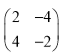has

Civil Engineering - (CE) 2018 GATE Paper (Practice Test - 2) - Question 37

The Laplace transform F (s ) of the exponential function,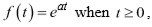where a is a constant and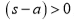, is

Civil Engineering - (CE) 2018 GATE Paper (Practice Test - 2) - Question 38

The rank of the following matrix is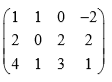Civil Engineering - (CE) 2018 GATE Paper (Practice Test - 2) - Question 39

Two rigid bodies of mass 5 kg and 4 kg are at rest on a frictionless surface until acted upon by a force of 36 N as shown in the figure. The contact force generated between the two bodies is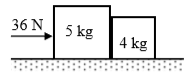Civil Engineering - (CE) 2018 GATE Paper (Practice Test - 2) - Question 40

Four bolts P, Q, R and S of equal diameter are used for a bracket subjected to a load of 130 kN as shown in the figure.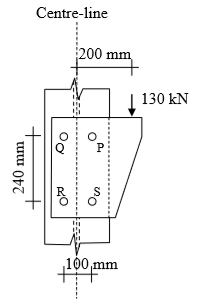The force in bolt P is

Civil Engineering - (CE) 2018 GATE Paper (Practice Test - 2) - Question 41

A singly-reinforced rectangular concrete beam of width 300 mm and effective depth 400 mm is to be designed using M25 grade concrete and Fe500 grade reinforcing steel. For the beam to be under-reinforced, the maximum number of 16 mm diameter reinforcing bars that can be provided is

Civil Engineering - (CE) 2018 GATE Paper (Practice Test - 2) - Question 42

A 3 m high vertical earth retaining wall retains a dry granular backfill with angle of internal friction of 30° and unit weight of 20 kN/m3. If the wall is prevented from yielding (no movement), the total horizontal thrust (in kN per unit length) on the wall is

Civil Engineering - (CE) 2018 GATE Paper (Practice Test - 2) - Question 43

Three soil specimens (Soil 1, Soil 2 and Soil 3), each 150 mm long and 100 mm diameter, are placed in series in a constant head flow set-up as shown in the figure. Suitable screens are provided at the boundaries of the specimens to keep them intact. The values of coefficient of permeability of Soil 1, Soil 2 and Soil 3 are 0.01, 0.003 and 0.03 cm/s, respectively.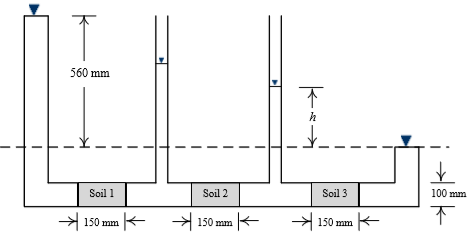The value of h in the set-up is

Civil Engineering - (CE) 2018 GATE Paper (Practice Test - 2) - Question 44

In a 5 m wide rectangular channel, the velocity u distribution in the vertical direction y is given by u = 1.25y1/6 . The distance y is measured from the channel bed. If the flow depth is 2 m, the discharge per unit width of the channel is

Civil Engineering - (CE) 2018 GATE Paper (Practice Test - 2) - Question 45

A car follows a slow moving truck (travelling at a speed of 10 m/s) on a two-lane two-way highway. The car reduces its speed to 10 m/s and follows the truck maintaining a distance of 16 m from the truck. On finding a clear gap in the opposing traffic stream, the car accelerates at an average rate of 4 m/s2, overtakes the truck and returns to its original lane. When it returns to its original lane, the distance between the car and the truck is 16 m. The total distance covered by the car during this period (from the time it leaves its lane and subsequently returns to its lane after overtaking) is

Civil Engineering - (CE) 2018 GATE Paper (Practice Test - 2) - Question 46

A level instrument at a height of 1.320 m has been placed at a station having a Reduced Level (RL) of 112.565 m. The instrument reads -2.835 m on a levelling staff held at the bottom of a bridge deck. The RL (in m) of the bottom of the bridge deck is

Civil Engineering - (CE) 2018 GATE Paper (Practice Test - 2) - Question 47

The value (up to two decimal places) of a line integral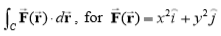along C which is a straight line joining (0,0) to (1,1) is ______

Civil Engineering - (CE) 2018 GATE Paper (Practice Test - 2) - Question 48

An 8 m long simply-supported elastic beam of rectangular cross-section (100 mm × 200 mm) is subjected to a uniformly distributed load of 10 kN/m over its entire span. The maximum principal stress (in MPa, up to two decimal places) at a point located at the extreme compression edge of a cross-section and at 2 m from the support is ______

Civil Engineering - (CE) 2018 GATE Paper (Practice Test - 2) - Question 49

A prismatic beam P-Q-R of flexural rigidity EI = 1x104 kNm2 is subjected to a moment of 180 kNm at Q as shown in the figure.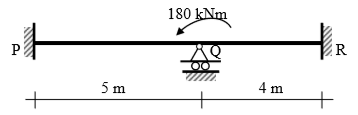A prismatic beam P-Q-R of flexural rigidity EI = 1x104 kNm2 is subjected to a moment of 180 kNm at Q as shown in the figure.

Civil Engineering - (CE) 2018 GATE Paper (Practice Test - 2) - Question 50

A prismatic propped cantilever beam of span L and plastic moment capacity M p is subjected to a concentrated load at its mid-span. If the collapse load of the beam is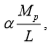the value of a is ______

Civil Engineering - (CE) 2018 GATE Paper (Practice Test - 2) - Question 51

A 6 m long simply-supported beam is prestressed as shown in the figure.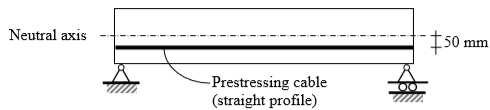The beam carries a uniformly distributed load of 6 kN/m over its entire span. If the effective flexural rigidity EI = 2×104 kNm2 and the effective prestressing force is 200 kN, the net increase in length of the prestressing cable (in mm, up to two decimal places) is ______

Civil Engineering - (CE) 2018 GATE Paper (Practice Test - 2) - Question 52

A cable PQ of length 25 m is supported at two ends at the same level as shown in the figure. The horizontal distance between the supports is 20 m. A point load of 150 kN is applied at point R which divides it into two equal parts.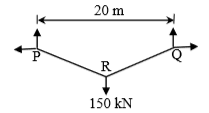Neglecting the self-weight of the cable, the tension (in kN, in integer value) in the cable due to the applied load will be ______

Civil Engineering - (CE) 2018 GATE Paper (Practice Test - 2) - Question 53

The compression curve (void ratio, e vs. effective stress,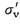) for a certain clayey soil is a straight line in a semi-logarithmic plot and it passes through the points (e = 1.2; σvʹ = 50 kPa) and (e = 0.6; σvʹ = 800 kPa). The compression index (up to two decimal places) of the soil is ______

Civil Engineering - (CE) 2018 GATE Paper (Practice Test - 2) - Question 54

The total horizontal and vertical stresses at a point X in a saturated sandy medium are 170 kPa and 300 kPa, respectively. The static pore-water pressure is 30 kPa. At failure, the excess pore-water pressure is measured to be 94.50 kPa, and the shear stresses on the vertical and horizontal planes passing through the point X are zero. Effective cohesion is 0 kPa and effective angle of internal friction is 36°. The shear strength (in kPa, up to two decimal places) at point X is ______

Civil Engineering - (CE) 2018 GATE Paper (Practice Test - 2) - Question 55

A group of nine piles in a 3 × 3 square pattern is embedded in a soil strata comprising dense sand underlying recently filled clay layer, as shown in the figure. The perimeter of an individual pile is 126 cm. The size of pile group is 240 cm × 240 cm. The recently filled clay has undrained shear strength of 15 kPa and unit weight of 16 kN/m3.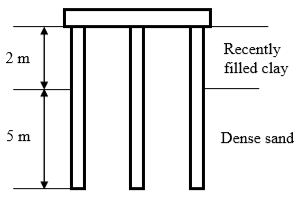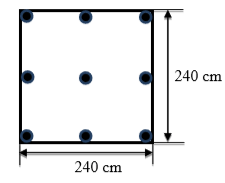The negative frictional load (in kN, up to two decimal places) acting on the pile group is ______

Civil Engineering - (CE) 2018 GATE Paper (Practice Test - 2) - Question 56

A three-fluid system (immiscible) is connected to a vacuum pump. The specific gravity values of the fluids (S1, S2) are given in the figure.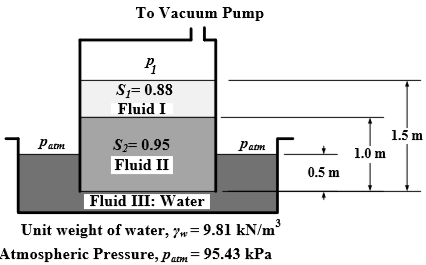The gauge pressure value (in kN/m2, up to two decimal places) of ��1 is ______

Civil Engineering - (CE) 2018 GATE Paper (Practice Test - 2) - Question 57

The total rainfall in a catchment of area 1000 km2, during a 6 h storm, is 19 cm. The surface runoff due to this storm computed from triangular direct run off hydrograph is 1×108 m3. The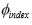index for this storm (in cm/h, up to one decimal place) is ______

Civil Engineering - (CE) 2018 GATE Paper (Practice Test - 2) - Question 58

A rough pipe of 0.5 m diameter, 300 m length and roughness height of 0.25 mm, carries water (kinematic viscosity = 0.9×10-6 m2/s) with velocity of 3 m/s. Friction factor (f) for laminar flow is given by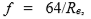and for turbulent flow it is given by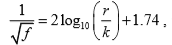where, Re = Reynolds number, r = radius of pipe, k = roughness height and g = 9.81 m/s2. The head loss (in m, up to three decimal places) in the pipe due to friction is ______

Civil Engineering - (CE) 2018 GATE Paper (Practice Test - 2) - Question 59

A flocculation tank contains 1800 m3 of water, which is mixed using paddles at an average velocity gradient G of 100/s. The water temperature and the corresponding dynamic viscosity are 30oC and 0.798×10-3 Ns/m2, respectively. The theoretical power required to achieve the stated value of G (in kW, up to two decimal places) is ______

Civil Engineering - (CE) 2018 GATE Paper (Practice Test - 2) - Question 60

A coal containing 2% sulfur is burned completely to ash in a brick kiln at a rate of 30 kg/min. The sulfur content in the ash was found to be 6% of the initial amount of sulfur present in the coal fed to the brick kiln. The molecular weights of S, H and O are 32, 1 and 16 g/mole, respectively. The annual rate of sulfur dioxide (SO2) emission from the kiln (in tonnes/year, up to two decimal places) is ______

Civil Engineering - (CE) 2018 GATE Paper (Practice Test - 2) - Question 61

At a small water treatment plant which has 4 filters, the rates of filtration and backwashing are 200 m3/d/m2 and 1000 m3/d/m2, respectively. Backwashing is done for 15 min per day. The maturation, which occurs initially as the filter is put back into service after cleaning, takes 30 min. It is proposed to recover the water being wasted during backwashing and maturation. The percentage increase in the filtered water produced (up to two decimal places) would be ______

Civil Engineering - (CE) 2018 GATE Paper (Practice Test - 2) - Question 62

A schematic flow diagram of a completely mixed biological reactor with provision for recycling of solids is shown in the figure.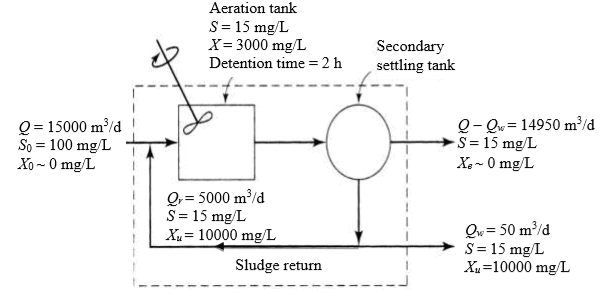Q, Qr, Qw = flow rates, m3/d
X0, X, Xe, Xu = microorganism concentrations (mixed-liquor volatile suspended solids or MLVSS), mg/L
The mean cell residence time (in days, up to one decimal place) is ______

Civil Engineering - (CE) 2018 GATE Paper (Practice Test - 2) - Question 63

The space mean speed (kmph) and density (vehicles/km) of a traffic stream are linearly related. The free flow speed and jam density are 80 kmph and 100 vehicles/km respectively. The traffic flow (in vehicles/h, up to one decimal place) corresponding to a speed of 40 kmph is ______

Civil Engineering - (CE) 2018 GATE Paper (Practice Test - 2) - Question 64

A 7.5 m wide two-lane road on a plain terrain is to be laid along a horizontal curve of radius 510 m. For a design speed of 100 kmph, super-elevation is provided as per IRC: 73-1980. Consider acceleration due to gravity as 9.81 m/s2. The level difference between the inner and outer edges of the road (in m, up to three decimal places) is ______

Civil Engineering - (CE) 2018 GATE Paper (Practice Test - 2) - Question 65

An aerial photograph of a terrain having an average elevation of 1400 m is taken at a scale of 1:7500. The focal length of the camera is 15 cm. The altitude of the flight above mean sea level (in m, up to one decimal place) is ______

## GATE Past Year Papers for Practice (All Branches)

407 docs|127 tests
Information about Civil Engineering - (CE) 2018 GATE Paper (Practice Test - 2) Page
In this test you can find the Exam questions for Civil Engineering - (CE) 2018 GATE Paper (Practice Test - 2) solved & explained in the simplest way possible. Besides giving Questions and answers for Civil Engineering - (CE) 2018 GATE Paper (Practice Test - 2), EduRev gives you an ample number of Online tests for practice

## GATE Past Year Papers for Practice (All Branches)

407 docs|127 tests(Scan QR code)